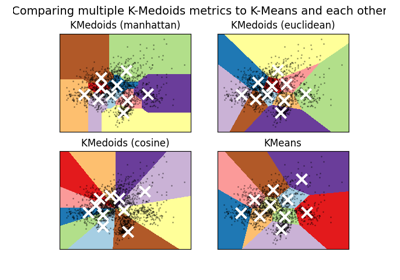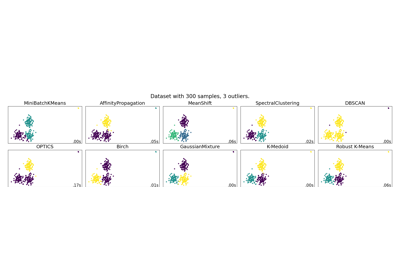# sklearn_extra.cluster.KMedoids¶

class sklearn_extra.cluster.KMedoids(n_clusters=8, metric='euclidean', method='alternate', init='heuristic', max_iter=300, random_state=None)[source]

k-medoids clustering.

Read more in the User Guide.

Parameters
n_clustersint, optional, default: 8

The number of clusters to form as well as the number of medoids to generate.

metricstring, or callable, optional, default: ‘euclidean’

What distance metric to use. See :func:metrics.pairwise_distances

method{‘alternate’, ‘pam’}, default: ‘alternate’

Which algorithm to use.

init{‘random’, ‘heuristic’, ‘k-medoids++’, ‘build’}, optional, default: ‘build’

Specify medoid initialization method. ‘random’ selects n_clusters elements from the dataset. ‘heuristic’ picks the n_clusters points with the smallest sum distance to every other point. ‘k-medoids++’ follows an approach based on k-means++_, and in general, gives initial medoids which are more separated than those generated by the other methods. ‘build’ is a greedy initialization of the medoids used in the original PAM algorithm. Often ‘build’ is more efficient but slower than other initializations on big datasets and it is also very non-robust, if there are outliers in the dataset, use another initialization.

max_iterint, optional, default

Specify the maximum number of iterations when fitting. It can be zero in which case only the initialization is computed which may be suitable for large datasets when the initialization is sufficiently efficient (i.e. for ‘build’ init).

random_stateint, RandomState instance or None, optional

Specify random state for the random number generator. Used to initialise medoids when init=’random’.

KMeans

The KMeans algorithm minimizes the within-cluster sum-of-squares criterion. It scales well to large number of samples.

Notes

Since all pairwise distances are calculated and stored in memory for the duration of fit, the space complexity is O(n_samples ** 2).

References

Maranzana, F.E., 1963. On the location of supply points to minimize

transportation costs. IBM Systems Journal, 2(2), pp.129-135.

Park, H.S.and Jun, C.H., 2009. A simple and fast algorithm for K-medoids

clustering. Expert systems with applications, 36(2), pp.3336-3341.

Examples

>>> from sklearn_extra.cluster import KMedoids
>>> import numpy as np

>>> X = np.asarray([[1, 2], [1, 4], [1, 0],
...                 [4, 2], [4, 4], [4, 0]])
>>> kmedoids = KMedoids(n_clusters=2, random_state=0).fit(X)
>>> kmedoids.labels_
array([0, 0, 0, 1, 1, 1])
>>> kmedoids.predict([[0,0], [4,4]])
array([0, 1])
>>> kmedoids.cluster_centers_
array([[1, 2],
[4, 2]])
>>> kmedoids.inertia_
8.0


See scikit-learn-extra/examples/plot_kmedoids_digits.py for examples of KMedoids with various distance metrics.

Attributes
cluster_centers_array, shape = (n_clusters, n_features)

or None if metric == ‘precomputed’

Cluster centers, i.e. medoids (elements from the original dataset)

medoid_indices_array, shape = (n_clusters,)

The indices of the medoid rows in X

labels_array, shape = (n_samples,)

Labels of each point

inertia_float

Sum of distances of samples to their closest cluster center.

__init__(n_clusters=8, metric='euclidean', method='alternate', init='heuristic', max_iter=300, random_state=None)[source]

Initialize self. See help(type(self)) for accurate signature.

## Examples using sklearn_extra.cluster.KMedoids¶